Please Support the Monkey! Tell All your Friends and Teachers
 ANSWER EXPLANATIONS Test Paper I : Section IV : Quantitative Section Do not try to get at the answer by performing calculations. It will only waste a tremendous amount of time. Analyze as follows: Consider the nos.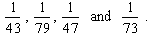The fact that the numerators are the same ( namely 1 ), indicates that the fraction with the greater denominator is the larger fraction. Hence,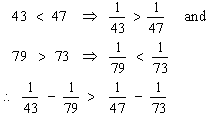Since the number in column A ( i.e. LHS ) is smaller than the number in column B, the smaller number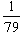(smaller compared to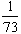) is subtracted from a larger number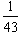(larger compared to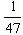) . Hence A. Whenever proper positive fractions are added, then their value becomes more than either one, but when they are multiplied, it becomes less than either one. e.g.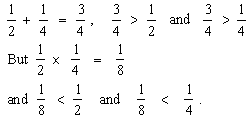Hence A. Test 1 Section 1 : Verbal Section Section 2 : Quantitative Section Section 3 : Analytical Section Section 4 : Quantitative Section Section 5 : Verbal Section Section 6 : Analytical Section Section 7 : Verbal Section Answer Key To Test 1 Answer Explanation To Test 1 Section 1 : Verbal Section Section 2 : Quantitative Section Section 3 : Analytical Section Section 4 : Quantitative Section Section 5 : Verbal Section Section 6 : Analytical Section Section 7 : Verbal Section